Article citation information:

Matyja, T. Tilt test of a pallet load unit – simulation studies. Scientific Journal of Silesian University of Technology. Series Transport. 2020, 107, 107-117. ISSN: 0209-3324. DOI: https://doi.org/10.20858/sjsutst.2020.107.8.

Tomasz MATYJA

TILT TEST OF A PALLET LOAD UNIT – SIMULATION STUDIES

Summary. This paper presents a dynamic model of a palletised load unit during a static tilt test. The stability (also called rigidity) of a load unit was evaluated. The palletised load unit was built of packages forming layers and protected against disintegration by stretch film. The aim of this study was to compare the results of a static tilt test with a commonly used and recommended dynamic acceleration test.

Keywords: tilt test, load unit, stability, simulation model

1. INTRODUCTION

Directive 2014/47/EU  and EUMOS 40509  recommend checking the stability of a load unit by means of dynamic tests, which may consist of appropriate rapid acceleration of the load (acceleration tests) or its stopping (crash tests). In both cases, access to specialised devices in the form of mobile platforms is required. Therefore, dynamic testing is expensive.

When there is no access to such devices, the stability of the load unit can also be assessed by performing a static test on an inclined plane . It is for this purpose that a rigid plate with dimensions enabling the pallet to be placed on it and a forklift to raise the end of the plate (Fig. 1). Tilt tests have another application; testing the stress relaxation and creep of the film stretch in time (endurance tests). This type of testing is not a subject of interest in this work.

This paper presents a simplified model of a pallet load unit with a layered structure during a quasi-static tilt test. Cargo layers shifts were limited by pre-stretched stretch film. The tilt test simulation results were compared with the simulation results of a typical dynamic test performed in accordance with standards [5, 12]. The developed mathematical model of the tilt test was implemented in the Matlab environment.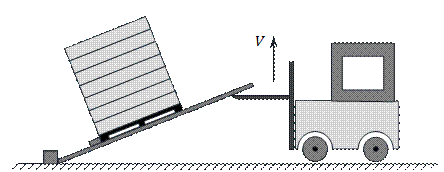Fig. 1. Performing a static test using a forklift

2. TILT TEST SIMULATION MODEL

During dynamic tests, the acceleration acting on the load unit should reach the value of 0.8g [5, 12]. This value of acceleration acting down the ramp can be obtained with a slope 53.13o. Of course, this is accompanied by a decrease in the force pressing the load unit perpendicular to the inclined plane. Therefore, it is difficult to directly compare the effectiveness of both dynamic and static tests. It seems expedient to consider the acceleration ratio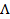, which is the quotient of the acceleration components parallel and perpendicular to the pallet plane. For the maximum acceleration value will be: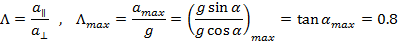(1)

Equation 1 shows that to achieve comparable conditions to the dynamic test, a tilt of the ramp at an angle 38.66o is necessary. When the lifting speed of the forklift is constant V=const, the height of the lift H(t)=Vt and based on the drawing (Fig.3), the angle of inclination of the ramp changes according to the relationship: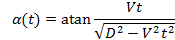(2)

At a typical value of forklift speed V=0.2m/s and plate length D=2m, the time needed to achieve the suitable angle of inclination is equal t=6.25s. Differences in acceleration ratio during dynamic and static tests are explained in Fig. 2.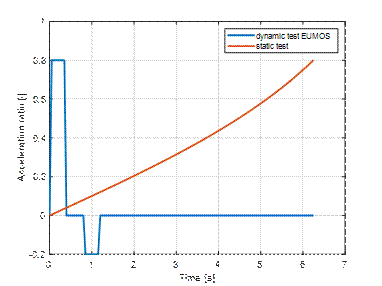Fig. 2. The time course of the acceleration ratio in dynamic and static tests

It can be assumed that the lifting process is slow enough to neglect the inertia forces associated with rotation in the cargo movement equations. This is confirmed by the considerations presented below. By differentiating twice formula for the sine of the angle a, we get: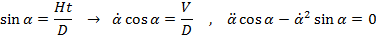(3)

And after transformations finally: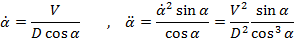(4)

When a changes from 0o to 60o then the angular velocity changes from V/D to 2V/D. At the same time, the angular acceleration varies from 0 to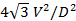. In addition to gravitational acceleration, the cargo will also be affected by inertial accelerations: centrifugal, tangential and Corilois acceleration. The maximum acceleration values in the anticipated platform rotation range will be, respectively: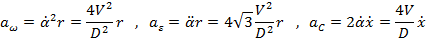(5)

At a low lifting speed